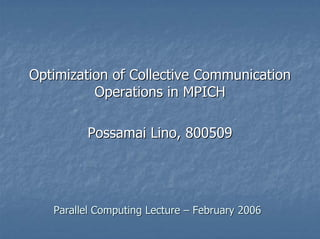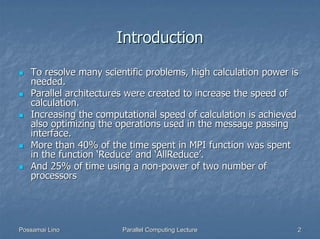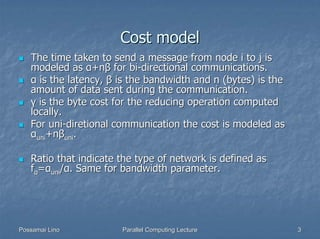Successfully reported this slideshow.

# Optimization of Collective Communication in MPICH

7

Share×
1 of 21
1 of 21

# Optimization of Collective Communication in MPICH

7

Share

This is a lecture about the paper: "Optimization of Collective Communication in MPICH". Department of Computer Science, University Ca' Foscari of Venice, Italy

This is a lecture about the paper: "Optimization of Collective Communication in MPICH". Department of Computer Science, University Ca' Foscari of Venice, Italy

## More Related Content

### Related Books

Free with a 14 day trial from Scribd

See all

### Related Audiobooks

Free with a 14 day trial from Scribd

See all

### Optimization of Collective Communication in MPICH

1. 1. Optimization of Collective Communication Operations in MPICH Possamai Lino, 800509 Parallel Computing Lecture – February 2006
2. 2. Introduction To resolve many scientific problems, high calculation power is needed. Parallel architectures were created to increase the speed of calculation. Increasing the computational speed of calculation is achieved also optimizing the operations used in the message passing interface. More than 40% of the time spent in MPI function was spent in the function ‘Reduce’ and ‘AllReduce’. And 25% of time using a non-power of two number of processors Possamai Lino Parallel Computing Lecture 2
3. 3. Cost model The time taken to send a message from node i to j is modeled as α+nβ for bi-directional communications. α is the latency, β is the bandwidth and n (bytes) is the amount of data sent during the communication. γ is the byte cost for the reducing operation computed locally. For uni-diretional communication the cost is modeled as αuni+nβuni. Ratio that indicate the type of network is defined as fα=αuni/α. Same for bandwidth parameter. Possamai Lino Parallel Computing Lecture 3
4. 4. *-to-all operations AllGather (all-to-all) Consist of gathering data from all nodes and distribute it to all Broadcast (one-to-all) Is a operation of broadcasting a data from a root node to every other node. All-to-all Each node send his unique data to every other processes. Different from allgather because the data owned by each node are not part of a unique vector Possamai Lino Parallel Computing Lecture 4
5. 5. Allgather The old algorithm uses a ring method. At each step (p-1 in total), node i send its data to node i+1 and receives data from i-1 (with wrap around). Actually used for large/medium messages and for non-power of two number of processes. A first optimization consist of using a recursive vector doubling with distance doubling technique as in figure. The amount of data sent by each process is 2kn/p, where k is the current step, ranging from 0 to log2 p - 1. So, the total cost is: α log2 p + nβ(p-1)/p Possamai Lino Parallel Computing Lecture 5
6. 6. Broadcast Binomial tree algorithm is the old algorithm used in MPICH. Good for short messages because of the latency term. Van Der Geijn has proposed an algorithm for long messages that takes a message, divide and scatter it between nodes and finally, collect them back to every node (allgather). The total cost is: [α log2 p + nβ(p-1)/p ] + [(p-1)α + nβ(p-1)/p]= α(log2 p + p - 1) + 2nβ(p- 1)/p. Possamai Lino Parallel Computing Lecture 6
7. 7. Reduce operations Reduce A root node computes a reduction function using the data gathered from all processes Reduce-scatter (all-to-all reduction) Is a reduction in which, at the end, the result vector is scattered between the processes AllReduce Is a reduction followed by a allgather of the resulting vector Possamai Lino Parallel Computing Lecture 7
8. 8. Terminology Recursive vector halving: the vector to be reduced is recursively halved in each step. Recursive vector doubling: small pieces of the vector scattered across processes are recursively gathered or combined to form the large vector Recursive distance halving: the distance over which processes communicate is recursively halved at each step (p / 2, p / 4, ... , 1). Recursive distance doubling: the distance over which processes communicate is recursively doubled at each step (1, 2, 4, ... , p / 2). Possamai Lino Parallel Computing Lecture 8
9. 9. Reduce-scatter operation 1/2 Old algorithm implement this operation as a binomial tree reduction to rank 0, followed by a linear scatterv. The total cost is the sum of the binomial tree reduction plus the cost of the linear scatterv, so (log2 p + p -1) α + (log2 p + (p-1)/p) nβ + (log2 p) nγ The choice for the best algorithm depends on the type of reduce operation: commutative or not-commutative For commutative reduce operation, and for short messages, recursive-halving algorithm is used. For not-commutative, recursive-doubling is used. Possamai Lino Parallel Computing Lecture 9
10. 10. Recursive-halving (commutative) Different implementations whether p is power of two or not. In the first case, log2 p steps are taken and on each of them bi-directional communication is performed. The data sent is halved at each step. Each process sends the data needed by all processes in the other half and receives the data needed by all processes in its own half In the second case, we reduce the number of processes to the nearest lower power of two, before applying the r-h algorithm to the rest of nodes. Finally, we distribute the data result vector to r=p-p’ processes excluded Possamai Lino Parallel Computing Lecture 10
11. 11. Recursive-doubling (non commutative) Similar to allgather optimized algorithm At each step k, from 0 to log2 p -1, processes communicate (n- n/p 2k) data Possamai Lino Parallel Computing Lecture 11
12. 12. Reduce-scatter for long messages Previous algorithms works well if the messages are short In other cases, pairwise exchange algorithm is used. Needed p-1 steps where in each step i, each process sends data to process (rank + i) and receives data from process (rank – i) and finally perform local reduction. Amount of data sent at each step n/p Same bandwidth requirement as the recursive halving algorithm Possamai Lino Parallel Computing Lecture 12
13. 13. Switching between algorithms as optimization Possamai Lino Parallel Computing Lecture 13
14. 14. Reduce Old algorithm use a binomial tree that takes log2 p steps Good for short messages but not best for long messages. They propose an optimized algorithm named Rabenseifner that utilizes less bandwidth. It is a reduce-scatter (recursive-halving) followed by a binomial tree gather to the root node. The cost is the sum of reduce- scatter and gather. Good for power of two number of processes. Possamai Lino Parallel Computing Lecture 14
15. 15. Reduce (non-power of two nodes) In this case, before using the above algorithm, we must arrange the number of processes. Reducing to the nearest lower power of two nodes is necessary, so p’=2‫ ﺎ‬log p ‫ﻟ‬ And the number of nodes removed is r=p-p’. The reduction is obtained combining half part of data of first 2r nodes to the same even ranked nodes. Finally, the first r nodes plus the last p-2r nodes are power of two and now we are able to apply po2 algorithms Reduction cost: (1-fα)α + (1+fβ)βn/2 + γn/2 Possamai Lino Parallel Computing Lecture 15
16. 16. Reduce/AllReduce npo2 schema Possamai Lino Parallel Computing Lecture 16
17. 17. AllReduce (power of two nodes) Used a recursive doubling algorithm for short and long messages and for user-defined reduction operations. For long and predefined reduction operation messages, Rebenseifnesr algorithm is used. Similar to reduce implementation, starts with a reduce-scatter and is followed by an allgather. Total cost: 2α log2 p + 2nβ(p-1)/p + nγ(p-1)/p Possamai Lino Parallel Computing Lecture 17
18. 18. AllReduce (non power of two nodes) Similar implementation of reduce, but after the reduction on power of two nodes, and after the recursive algorithm, follow an allgather operation. Allgather implemented using a recursive vector doubling and distance halving for the first r+(2r-p) nodes. For the other r nodes (p-r+2r-p), we need an additional overhead for sending the result data. This step takes αuni+nβuni Possamai Lino Parallel Computing Lecture 18
19. 19. Results comparison Vendor MPI/ newer MPI implementation Older/newer implementation of operations Possamai Lino Parallel Computing Lecture 19
20. 20. Index Introduction Cost Model *-to-all operations Allgather Broadcast Reduce operations Terminology Reduce-scatter Reduce AllReduce Results comparison Possamai Lino Parallel Computing Lecture 20
21. 21. References Thakur, Rabenseifner, Gropp Optimization of Collective Communication Operations in MPICH The Int. J. of High Performance Computing Applications, Volume 19, No. 1, Spring 2005, pp. 49–66. Possamai Lino Parallel Computing Lecture 21